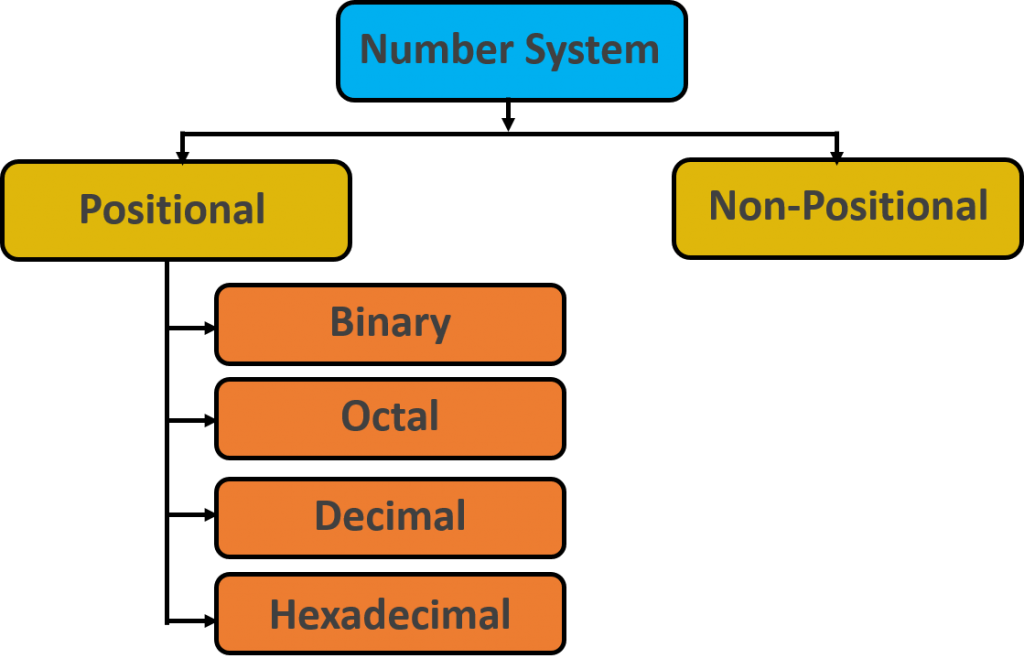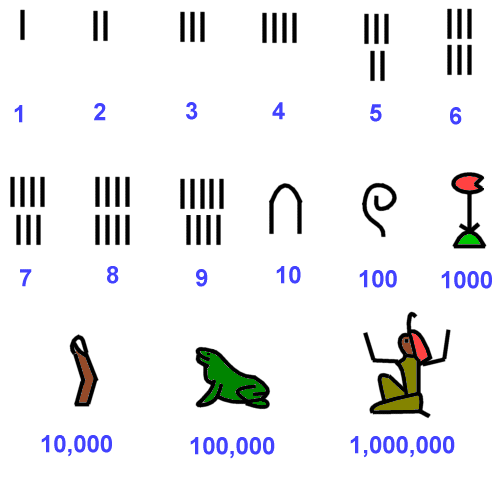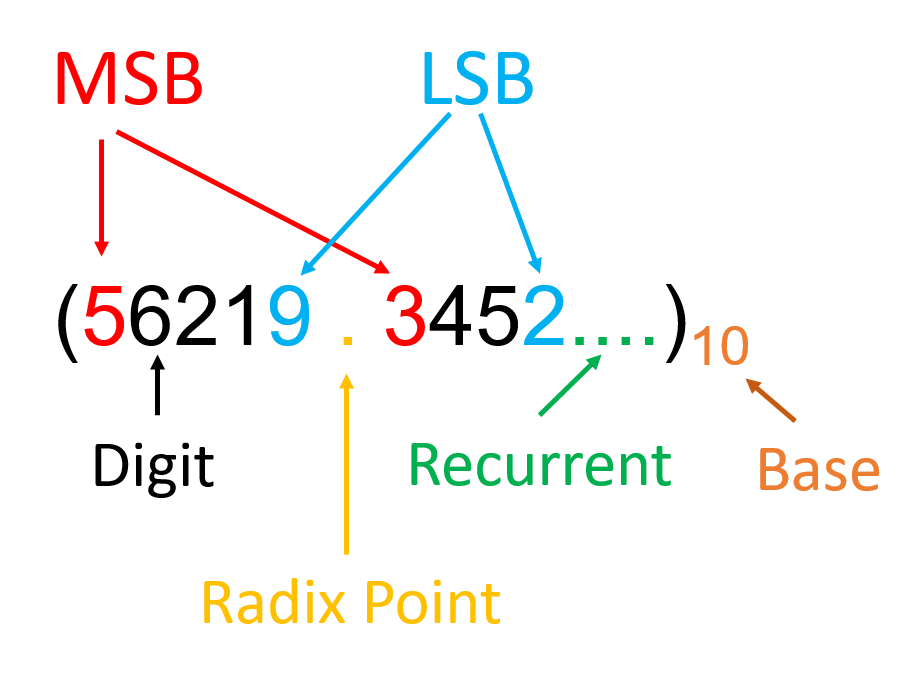# Third Chapter Lesson-1: Concept of Number System and Its’ Classification.

## At the end of this lesson-

• 1. You will be able to describe the history of number.
• 2. You will be able to differentiate number and digit.
• 3. You will be able to describe the types of number system.
• 4. You will be able to differentiate different types of number systems.

## Go For Bangla Version

Number: A number is a string of one or more digits which is used to count, quantify and measure something. For example, there are 20 students in a class ; Here 20 is a number.

Digit: A digit is a single numerical symbol of a number or the smallest symbols of numbers. All digits are numbers but all numbers are not digit. For example, 243 is a number with three digits, which is consist of 2, 3 and 3 separate three digits. Each of them is a separate number.

The value of each digit in a number can be determined using −

• The digit
• The position of the digit in the number
• The base of the number system

Number system: The technique to represent and work with numbers is called number system. Decimal number system is the most common number system.

The number system consists of the following elements. As-

• Some symbols- For example- 1,2,3 …
• Some operators. For example –  +, -, ×, ÷ etc.
• Some rules. For example, the rules of addition, subtraction, multiplication, division etc.

## Types of Number system:Number system is classified in two ways based on the position of the digits in a number. They are-

• Non-Positional number system
• Positional number system

## Non-Positional number system:

In Non-Positional number system, each symbol represents the same value regardless of its position.

The number system in which the value of a number does not depend on the position of the digits used in the number is called the non-positional number system. In this system, position of the symbols or digits does not matter. As a result, the digits have no local value. On the basis of its own value, the calculation is done by whom. Hieroglyphics, Mayan and Roman used in ancient times, are an example of a non-positional number system.Fig: Hieroglyphics number system

## Positional number system:

In Positional number system, each symbol represents different value depending on the position they occupy in a number.

The number system in which the value of a number depends on the position of the digits used in the number is called the positional number system. In this number system, value of a number is calculated using local value of the digits, positional value of the digits and base of the number system. In this system, Each number is divided into integer and fraction with Radix point (.). For example: (126.34)10

In any positional number system,

The Positional value of a digit in a number is = (the base of the number)the position of the digit .

the position of the digits of a integer number starts from 0 (right to left) and the position of the digits of a fractional number starts from -1 (left to right). For example,a number (126.34)10; the positional value of the digit 2 is (10)1 = 10 and the positional value of the digit 1 is (10)2 = 100.

Different parts of a number in positional number system-## Types of Positional number system:

Positional number system is four types. They are-

• Binary
• Octal
• Decimal

### Binary Number System:

A Binary number system has only two digits that are 0 and 1. Every number (value) represents with 0 and 1 in this number system. The base of binary number system is 2, because it has only two digits.

It is also called as base 2 number system. The easiest way to vary instructions through electric signals is two-state system – on and off. ON is represented as 1 and OFF as 0, though 0 is not actually no signal but signal at a lower voltage.

Each binary digit is also called a bit. Binary number system is also positional number system, where each digit has a value expressed in powers of 2.

In any binary number, the rightmost digit is called least significant bit (LSB) and leftmost digit is called most significant bit (MSB).

Computer memory is measured in terms of how many bits it can store. Here is a chart for memory capacity conversion.

• 1 byte (B) = 8 bits
• 1 Kilobytes (KB) = 1024 bytes
• 1 Megabyte (MB) = 1024 KB
• 1 Gigabyte (GB) = 1024 MB
• 1 Terabyte (TB) = 1024 GB
• 1 Exabyte (EB) = 1024 PB
• 1 Zettabyte = 1024 EB
• 1 Yottabyte (YB) = 1024 ZB

### Octal Number System:

Octal number system has only eight (8) digits from 0 to 7. Every number (value) represents with 0,1,2,3,4,5,6 and 7 in this number system. The base of octal number system is 8, because it has only 8 digits.

Octal number system is also a positional number system with where each digit has its value expressed in powers of 8.It is also called as base 8 number system.

### Decimal Number System:

Decimal number system has only ten (10) digits from 0 to 9. Every number (value) represents with 0,1,2,3,4,5,6, 7,8 and 9 in this number system. The base of decimal number system is 10, because it has only 10 digits.

Decimal number system is also a positional Number system. This means that the value of digits will depend on its position.

A Hexadecimal number system has sixteen (16) alphanumeric values from 0 to 9 and A to F. Every number (value) represents with 0,1,2,3,4,5,6, 7,8,9,A,B,C,D,E and F in this number system. The base of hexadecimal number system is 16, because it has 16 alphanumeric values.

Here A is equal to 10, B is equal to 11, C is equal to 12, D is equal to 13, E is equal to 14 and F is equal to 15.

Hexadecimal number system is also a positional number system with where each digit has its value expressed in powers of 16.

### Base of the number system:

• Base of a number system is the total number of digits used in that number system.
• Base is used as subscript of a number.
• Number system with base ‘b’ has its digits in the range [0 , b-1].
• It is also called as radix of a number system.

Table of the Numbers Systems with Base, Used Digits, Representation:

## Lesson Evaluation-

Knowledge Based Questions:

• a. What is number?
• a. What is digit?
• a. What is bit/byte?
• a. What is number system?
• a. What is positional number system?
• a. What is Radix point?
• a. What is positional value?
• a. What is non-positional number system?
• a. What is binary number system?
• a. What is decimal number system?
• a. What is octal number system?
• a. What is hexadecimal number system?
• a. What is the base of number system?

Comprehension Based Questions:

• b. “All digits are numbers but all numbers are not digit” – explain.
• b. “Tally a non-positional number system” – Explain.
• b. Why the base of hexadecimal number system is 16? Explain.
• b. What is meant by the base of the number system? Explain it.
• b. Why the number (11)10 is called positional number?
• b. Explain the positional value of decimal number system.
• b. What type of number is 10101 in number system? Explain.
• b. What type of number is 988 in number system? Explain.
• b. What type of number is 3D? Explain.
• b. Explain 3-based number system.
• b. Is (298)8 valid number? –Explain.
• b. Binary number system is similar to the functions of internal parts of the computer. Explain.
• b. Why digital signal is significant in computer system? Explain.
• b. Write down the reasons for using binary number system in computer design.
• b. Computer cannot understand (169)10 Explain it.
• b. How will you differentiate decimal and hexadecimal number systems?
• b. “Octal number system is called 3-bit code” -Explain.
• b. “Hexadecimal number system is called 4-bit code” -Explain.

Creative Questions:

Multiple Choice Questions:

1. Which one is non-positional number system?

a) Binary     b) Hieroglyphics    c) Decimal      d) Octal

2. Computer uses normally which number system?

a) Binary     b) Octal       c) Decimal      d) Hexadecimal

3. How many digits  are in hexadecimal number system?

a) 6     b) 8      c) 10       d) 16

4. What is the base of Hexadecimal Number System?

a) 6     b) 8      c) 10       d) 16

5. What is the base of Decimal Number System?

a) 6     b) 8      c) 10       d) 16

6. What is the base of Binary Number System?

a) 2     b) 8      c) 10       d) 16

7. Which type of number is A68B in number system?

a) Binary     b) Octal       c) Decimal      d) Hexadecimal

8. How many types of Number system based on positional value?

a) 2     b) 4      c) 10       d) 16

9. Which one is the Smallest Unit for measurement of Memory?

a) Bit    b) Byte    c) Kilo Byte    d) Mega Byte

10. Which type of number is 101B in number system?

a) Binary     b) Octal       c) Decimal      d) Hexadecimal

11. What is indicated by 0 in (1110)2 ?

a) BOS      b) BCD      c) LSB        d) MSB

12. How many bits are in 1011?

a) 2      b) 3       c) 4     d) 10

13. Which type of number is 2BAD.8C  in number system?

a) Binary     b) Octal       c) Decimal      d) Hexadecimal

14. To determine the value of a positional number It needs–

i. Base of the Number    ii. Local value of digits        iii. Positional value of digits

Which one is correct?

a) i & ii        b) i & iii       c) ii & iii      d) i,ii & iii

15. The properties of Binary number system are-

i. Used as digital signal    ii. Understandable in Computer      iii. Base of computer’s calculations

Which one is correct?

a) i & ii        b) i & iii       c) ii & iii      d) i,ii & iii

16. Binary Number system is used in computer system depending on the properties are–

i. On, Off      ii. High, Low      iii. Positive, Negative

Which one is correct?

a) i & ii        b) i & iii       c) ii & iii      d) i,ii & iii

17. The number 549 can be –

i. Octal   ii. Decimal    iii. Hexadecimal

Which one is correct?

a) i & ii        b) i & iii       c) ii & iii      d) i,ii & iii

18. The number 310.76 can be –

i. Octal   ii. Decimal    iii. Hexadecimal

Which one is correct?

a) i & ii        b) i & iii       c) ii & iii      d) i,ii & iii

19. The number 111 can be –

i. Binary   ii. Decimal    iii. Hexadecimal

Which one is correct?

a) i & ii        b) i & iii       c) ii & iii      d) i,ii & iii

20. The number 678 can be –

i. Octal    ii. Decimal    iii. Hexadecimal

Which one is correct?

a) i & ii        b) i & iii       c) ii & iii      d) i,ii & iii

Written by,